JMSLTM Numerical Library 7.2.0
com.imsl.math

## Class ZerosFunction

• All Implemented Interfaces:
Serializable, Cloneable

```public class ZerosFunction
extends Object
implements Serializable, Cloneable```
Finds the real zeros of a real, continuous, univariate function, f(x).

`ZerosFunction` computes n real zeros of a real, continuous, univariate function f. The search for the zeros of the function can be limited to a specified interval, or extended over the entire real line. The algorithm is generally more efficient if an interval is specified. The user supplied function, f(x), must return valid results for all values in the specified interval. If no interval is given, the user-supplied function must return valid results for all real numbers.

The function has two convergence criteria. The first criterion accepts a root, x, if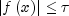where= `error`, see method `setError`.

The second criterion accepts a root if it is known to be inside of an interval of length at most `errorAbsolute`, see method `setAbsoluteError`.

A root is accepted if it satisfies either criteria and is not within `minSeparation` of another accepted root, see method `setMinimumSeparation`.

If initial guesses for the roots are given, Müller's method (Müller 1956) is used for each of these guesses. For each guess, the Müller iteration is stopped if the next step would be outside of the bound, if given. The iteration is also stopped if the algorithm cannot make further progress in finding a root.

If no guesses for the zeros were given, or if Müller's method with the guesses did not find the requested number of roots, a meta-algorithm, combining Müller's and Brent's methods, is used. Müller's method is used primarily to find the roots of functions, such as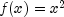, where the function does not cross the y=0 line. Brent's method is used to find other types of roots.

The meta-algorithm successively refines the interval using a one-dimensional Faure low-discrepancy sequence. The Faure sequence may be scaled by setting the bound interval [a,b] using the `setBounds` method. The Faure sequence will be scaled from (0,1) to (a,b).

If no bound on the function's domain is given, the entire real line must be searched for roots. In this case the Faure sequence is scaled from (0, 1) to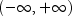using the mapping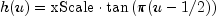where `xScale` is set by the `setXScale` method.

At each step of the iteration, the next point in the Faure sequence is added to the list of breakpoints defining the subintervals. Call the points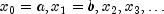. The new point,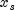splits an existing subinterval,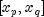.

The function is evaluated at. If its value is small enough, specifically if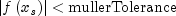then Müller's method is used with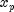,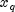andas starting values. If a root is found, it is added to the list of roots. If more roots are required, the new Faure point is used.

If Müller's method did not find a root using the new point, the function value at the point is compared with the function values at the endpoints of the subinterval it divides. If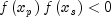and no root has previously been found in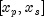, then Brent's method is used to find a root in this interval. Similarly, if the function changes sign over the interval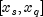, and a root has not already been found in the subinterval, Brent's method is used.

Example, Serialized Form
• ### Nested Class Summary

Nested Classes
Modifier and Type Class and Description
`static interface ` `ZerosFunction.Function`
Public interface for the user supplied function to `ZerosFunction`.
• ### Constructor Summary

Constructors
Constructor and Description
`ZerosFunction()`
Creates an instance of the solver.
• ### Method Summary

Methods
Modifier and Type Method and Description
`boolean` `allConverged()`
Returns true if the iterations for all of the roots have converged.
`double[]` `computeZeros(ZerosFunction.Function objectF)`
Returns the zeros of a univariate function.
`int` `getMaxEvaluations()`
Returns the maximum number of function evaluations allowed.
`int` `getNumberOfEvaluations()`
Returns the actual number of function evaluations performed.
`int` `getNumberOfRoots()`
Returns the requested number of roots to be found.
`int` `getNumberOfRootsFound()`
Returns the number of zeros found.
`void` `setAbsoluteError(double errorAbsolute)`
Sets the second convergence criterion.
`void` ```setBounds(double lowerBound, double upperBound)```
Sets the closed interval in which to search for the roots.
`void` `setError(double error)`
Sets the first convergence criterion.
`void` `setGuess(double[] guess)`
Sets the initial guess for the zeros.
`void` `setMaxEvaluations(int maxEvaluations)`
Sets the maximum number of function evaluations allowed.
`void` `setMinimumSeparation(double minSeparation)`
Sets the minimum separation between accepted roots.
`void` `setMullerTolerance(double mullerTolerance)`
Sets the tolerance used during refinement to determine if Müllers method is started.
`void` `setNumberOfRoots(int numRoots)`
Sets the number of roots to be found.
`void` `setXScale(double xScale)`
Sets the the scaling in the x-coordinate.
• ### Methods inherited from class java.lang.Object

`clone, equals, finalize, getClass, hashCode, notify, notifyAll, toString, wait, wait, wait`
• ### Constructor Detail

• #### ZerosFunction

`public ZerosFunction()`
Creates an instance of the solver.
• ### Method Detail

• #### allConverged

`public boolean allConverged()`
Returns true if the iterations for all of the roots have converged.
• #### computeZeros

`public double[] computeZeros(ZerosFunction.Function objectF)`
Returns the zeros of a univariate function.
Parameters:
`objectF` - contains the function for which the zeros will be found
Returns:
a `double` array containing the zerso of the univariate function.
• #### getMaxEvaluations

`public int getMaxEvaluations()`
Returns the maximum number of function evaluations allowed.
Returns:
an `int` containing the maximum number of function evaluations allowed
• #### getNumberOfEvaluations

`public int getNumberOfEvaluations()`
Returns the actual number of function evaluations performed.
Returns:
an `int` containing the actual number of function evaluations performed
• #### getNumberOfRoots

`public int getNumberOfRoots()`
Returns the requested number of roots to be found.
Returns:
an `int` containing the requested number of roots to be found
• #### getNumberOfRootsFound

`public int getNumberOfRootsFound()`
Returns the number of zeros found.
Returns:
an `int` containing the number of roots found
• #### setAbsoluteError

`public void setAbsoluteError(double errorAbsolute)`
Sets the second convergence criterion.

The second criterion accepts a root if the root is known to be inside an interval of length at most `errorAbsolute`.

Parameters:
`errorAbsolute` - a `double` value specifying the second convergence criterion. A root is accepted if the absolute value of the function at the point is less than or equal to `errorAbsolute`. `errorAbsolute` must be greater than or equal to 0.0. Default: `errorAbsolute` = 2.22e-14
• #### setBounds

```public void setBounds(double lowerBound,
double upperBound)```
Sets the closed interval in which to search for the roots. The function must be defined for all values in this interval.
Parameters:
`lowerBound` - a `double` containing the lower interval bound. `lowerBound` cannot be greater than or equal to `upperBound`.
`upperBound` - a `double` containing the upper interval bound.

By default the search for the roots is not bounded.

• #### setError

`public void setError(double error)`
Sets the first convergence criterion.

A root is accepted if it is bracketed within an interval of length `error`.where= `error`.

Parameters:
`error` - a `double` containing the first convergence criterion. `error` must be greater than or equal to 0.0. By default, `error` = 2.0e-8/`xScale`.
• #### setGuess

`public void setGuess(double[] guess)`
Sets the initial guess for the zeros.
Parameters:
`guess` - a `double` array containing the initial guesses for the number of zeros to be found. If a bound on the zeros is also given, the guesses must satisfy the bound condition.
• #### setMaxEvaluations

`public void setMaxEvaluations(int maxEvaluations)`
Sets the maximum number of function evaluations allowed.

Methods `allConverged` and `getNumberOfRootsFound` can be used to confirm whether or not the number of roots requested were found within the maximum evaluations specified. `maxEvaluations` must be greater than or equal to 0.0.

Parameters:
`maxEvaluations` - an `int` containing the maximum number of function evaluations allowed. Once this limit is reached, the roots found are returned.
• #### setMinimumSeparation

`public void setMinimumSeparation(double minSeparation)`
Sets the minimum separation between accepted roots.
Parameters:
`minSeparation` - a `double` containing the minimum separation between accepted roots. If two points satisfy the convergence criteria, but are within `minSeparation` of each other, only one of the roots is accepted. `minSeparation` must be greater than or equal to 0.0.

By default, `minSeparation` = 1.0e-8/`xScale`.

• #### setMullerTolerance

`public void setMullerTolerance(double mullerTolerance)`
Sets the tolerance used during refinement to determine if Müllers method is started.

Müller's method is started if, during refinement, a point is found for which the absolute value of the function is less than `mullerTolerance` and the point is not near an already discovered root. If `mullerTolerance` is less than or equal to zero, Müller's method is never used.

Parameters:
`mullerTolerance` - a `double` containing the tolerance used during refinement to determine when the Müller's method is used. By default, `mullerTolerance` = 1.0e-8/`errorAbsolute`
• #### setNumberOfRoots

`public void setNumberOfRoots(int numRoots)`
Sets the number of roots to be found.
Parameters:
`numRoots` - an `int` containing the number of roots to be found. `numRoots` must be greater than or equal to zero. By default, `numRoots`=1.
• #### setXScale

`public void setXScale(double xScale)`
Sets the the scaling in the x-coordinate.

If no bound on the function's domain is given, the entire real line must be searched for roots. In this case the Faure sequence is scaled from (0, 1) to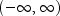using the mapping.
Parameters:
`xScale` - a `double` containing the scaling in the x-coordinate. The absolute value of the roots divided by `xScale` should be about one. `xScale` must be greater than 0.0. By default, `xScale`=1.0.
JMSLTM Numerical Library 7.2.0